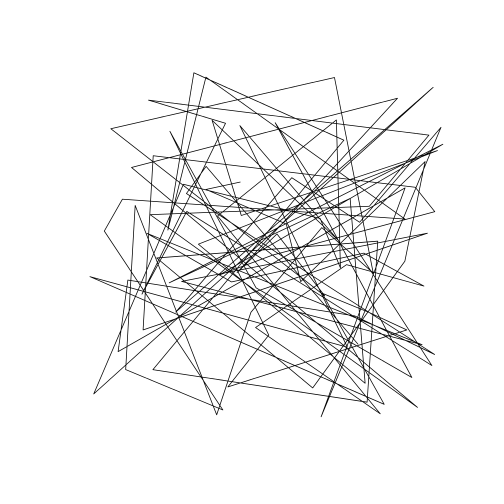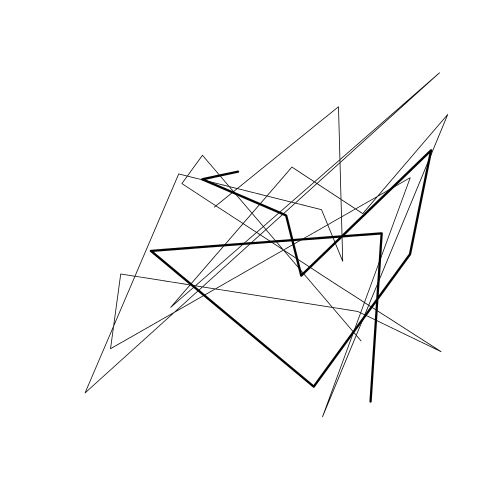# Introduction to spatial lines in R

This activity will introduce you to working with spatial lines in R.

R Skill Level: Intermediate - this activity assumes you have a working knowledge of `R`

# Objectives & Goals

Upon completion of this activity, you will:
• Know how to create and write spatial lines
• Know how to convert points to lines
• Be able to calculate distances along spatial lines

### Required packages

To complete the following activity you will need the following packages installed: raster sp rgeos

#### Installing the packages

If installing these packages for the first time consider adding `dependencies=TRUE`
• `install.packages("raster")`
• `install.packages("sp")`
• `install.packages("rgeos")`

• Last modified: 2019-09-20 18:26:28

``````library(raster)
library(sp)
library(geosphere)
library(rgeos)
``````

Creating spatial lines using the `sp` package is similar to making polygons from scratch - tedious. Here, for completeness we’ll make a few spatial lines from scratch. Once we know how to do that, we’ll use a simplier method.

To construct lines we first need to start with the locations of the endpoints. For this example, we’ll use the 100 random points we used for the SpatialPoints lession. Let’s also add an `ID` field as well.

``````# generate 100 random XY coords
x_coords <- runif(n = 100, min = -100, max = -80)
y_coords <- runif(n = 100, min = 25, max = 45)

# make a unique identifier for each line
ID <- paste0("line_",1:100)
``````
``````##    x_coords y_coords     ID
## 1 -91.69127 38.72604 line_1
## 2 -93.57623 38.32013 line_2
## 3 -89.15693 36.41894 line_3
## 4 -88.36280 33.25023 line_4
## 5 -81.52341 39.85124 line_5
## 6 -82.63692 34.37538 line_6
``````

Now that we have the endpoints, we need to create a `Line` object then convert that to a `Lines` object and give them an ID then we can finally convert them to `SpatialLines`. Here, we’ll use the first two points for our first line.

``````line_obj <- sp::Line(cbind(x_coords[1:2],y_coords[1:2]))

lines_obj <- sp::Lines(list(line_obj),ID=ID)

firstLine <- sp::SpatialLines(list(lines_obj))
``````

The code to generate a series of lines from a list of points gets a bit ugly and creating lines from scratch isn’t common - at least I haven’t done it very often in my work. Here are a few ‘easier’ ways to create `SpatialLine` objects.

First using the `sp` package again. Here, we’ll convert to `SpatialPoints` then use the `as` function to convert a `SpatialPoints` into a `SpatialLine`

``````# make SpatialPoints
points <- sp::SpatialPoints(cbind(x_coords,y_coords))

# use as to convert to line
sp_line <- as(points,"SpatialLines")
``````
``````## class       : SpatialLines
## features    : 1
## extent      : -99.9518, -80.54837, 25.81913, 44.73302  (xmin, xmax, ymin, ymax)
## crs         : NA
``````#### One your own:

How would you create a line with only the first 10 points instead of all of them? How would you make a line that included only the last 20 points?### Distance / length

Calculating the distance along a line / length of a line can be done using the `rgeos` package. It can also be done with the `sf` package. First, we’ll use the `gLength` function. We need to be a little careful here because the units it returns depends on the `coordinate reference system` the data have. See projections for details.

``````# returns length in coordinate reference system units
# here it's degrees - it assumes planar coordinates
rgeos::gLength(sp_line)
``````
``````##  990.6532
``````

Another option is to return the great circle distance. The following code calculates the great circle distance along a line and returns the distance in meters.

``````# great circle distance along our line
geosphere::lengthLine(sp_line)
``````
``````##  98616344
``````

#### One your own:

Find the great circle length in kilometers of a line that contains the first 25 locations.

Back to top